# Linearized Michaelis-Menten Equations

library(renz)
library(knitr)
data(ONPG, package = "renz")

We start by loading some kinetic data obtained by students during their undergraduate laboratory training. Using $$\beta$$-galactosidase as an enzyme model, the students assess the effect of the substrate o-nitrophenyl-$$\beta$$-D-galactopynaroside (ONPG) on the initial rate (doi: 10.1002/bmb.21522). The data obtained by eight different groups of students can be loaded just typing:

kable(ONPG)
ONPG v1 v2 v3 v4 v5 v6 v7 v8
0.05 2.26 1.29 0.004 0.004 0.004 0.003 1.77 2.98
0.10 5.48 3.33 0.008 0.007 0.007 0.006 5.20 5.20
0.25 13.40 11.80 0.020 0.020 0.016 0.017 15.04 14.38
0.50 24.70 22.80 0.035 0.035 0.032 0.031 28.31 30.30
1.00 40.90 35.20 0.060 0.056 0.050 0.048 50.98 48.99
2.50 62.30 39.90 0.110 0.104 0.090 0.101 75.42 86.25
5.00 94.30 73.50 0.138 0.138 0.115 0.121 112.68 112.57
8.00 105.00 12.90 0.154 0.150 0.119 0.139 126.06 136.24
20.00 133.00 112.00 0.179 0.179 0.142 0.152 154.93 169.97
30.00 144.00 120.00 0.200 0.200 0.166 0.181 168.75 177.71

The first column gives the ONPG concentrations in mM, and the remaining 8 columns correspond to the initial rates. Note that while groups 1, 2, 7 and 8 decided to express their rates as $$\mu$$M/min, the remaining groups opted by mM/min. This information can be confirmed by checking the attributes of data:

attributes(ONPG)
#> $names #>  "ONPG" "v1" "v2" "v3" "v4" "v5" "v6" "v7" "v8" #> #>$row.names
#>    1  2  3  4  5  6  7  8  9 10
#>
#> $[ONPG] #>  "mM" #> #>$v3, v4, v5, v6
#>  "mM/min"
#>
#> $class #>  "data.frame" #> #>$v1, v2, v7, v8
#>  "uM/min"

Thus, before continuing we are going to express all the rates using the same units: $$\mu$$M/min:

ONPG[ , 4:7] <- 1000 * ONPG[ , 4:7]

## First thing first: scatter plot

I strongly insist to my students that when we have to analyze data, the first thing we must do is a scatter diagram, since this will give us a first impression about our data and will guide us on how to proceed with the analysis. To lead by example, we will carry out such diagrams.

The first four groups:

oldmar <- par()$mar oldmfrow <- par()$mfrow
par(mfrow = c(2, 2))
par(mar = c(4, 4,1,1))
for (i in 2:5){
plot(ONPG$ONPG, ONPG[, i], ty = 'p', ylab = 'v (uM/min)', xlab = '[ONPG] (mM)') }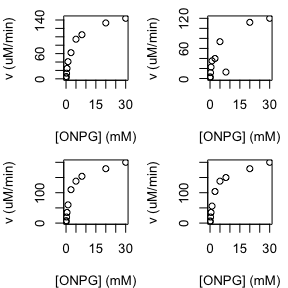par(mar = oldmar) par(mfrow = oldmfrow) The next four groups: oldmar <- par()$mar
oldmfrow <- par()$mfrow par(mfrow = c(2, 2)) par(mar = c(4, 4,1,1)) for (i in 6:9){ plot(ONPG$ONPG, ONPG[, i],
ty = 'p', ylab = 'v (uM/min)', xlab = '[ONPG] (mM)')
}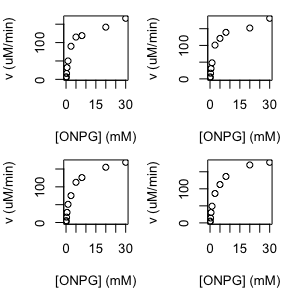par(mar = oldmar)
par(mfrow = oldmfrow)

In general, the data look OK. That is, the relationship between the dependent variable (initial rate) and the independent variable ([ONPG]) is what we expect: hyperbolic curve. An exception is the rate obtained by group 2 when [ONPG] = 8 mM, which is clearly an “outlier”. No problem! We will remove that point from further analysis to prevent it from introducing artifacts.

ONPG$v2 <- NA ## Linearizing the Michaelis-Menten equation The package renz provides four functions to obtain $$K_m$$ and $$V_{max}$$ using linear fitting of linearized Michaelis-Menten equations: • lb() (Lineweaver-Burk). • hw() (Hanes-Woolf). • eh() (Eadie-Hofstee). • ecb() (Eisenthal & Cornish-Bowden). ### Lineweaver-Burk Starting from the Michaelis-Menten equation and taking the inverses in both sides, one can obtain: $\begin{equation} \tag{1} \frac{1}{v} = \frac{K_m}{V_{max}} \frac{1}{[S]} + \frac{1}{V_{max}} \end{equation}$ Thus, to analyze our data using this equation we need to take the inverse of the substrate concentration and the inverse of the initial rate and fit them to the equation of a line. This can be achieved using the lb() function. For group 1: g1 <- lb(ONPG[ , c(1,2)])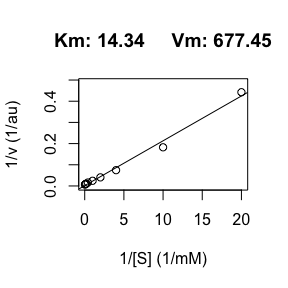For group 5: g5 <- lb(ONPG[ , c(1,6)])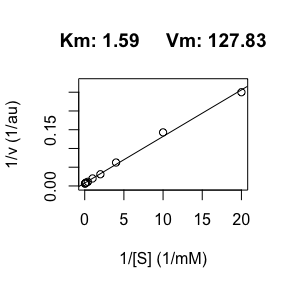For group 7: g8 <- lb(ONPG[ , c(1,8)])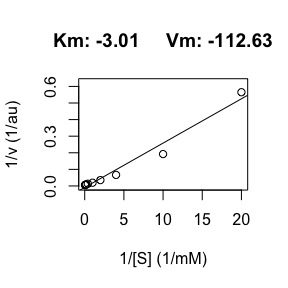These results seem to be quite perplexing. It looks like if each group had used a different enzyme! Even worse, group 7 obtains negative $$K_m$$ and $$V_{max}$$. These results are frustrating for the students, and an inexperienced instructor might be tempted to justify them appealing to a lack of skill of the student to generate precise data, which would be an awful mistake since, as we will show next, the quality of data from all the groups, including group 7, is good enough to obtain a reliable estimate of the β-galactosidase kinetic parameters when the analysis is properly carried out. To this end, all we have to do is to carry out a weighted linear regression of data, as it was suggested originally by Lineweaver and Burk (for details, see doi = 10.1002/bmb.21522). Now, let’s re-examine the data introducing weighted regression. For group 1: wg1 <- lb(ONPG[ , c(1,2)], weighting = TRUE)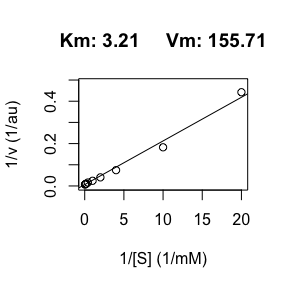For group 5: wg5 <- lb(ONPG[ , c(1,6)], weighting = TRUE)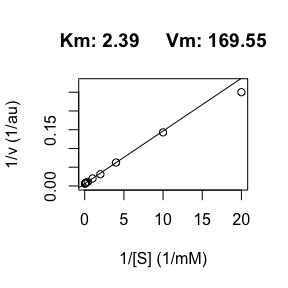For group 7: g7 <- lb(ONPG[ , c(1,8)], weighting = TRUE)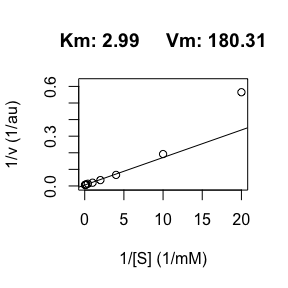As it can be observed, after analyzing the data using weighted regression, all the groups obtain similar estimates of $$K_m$$ and $$V_{max}$$, which does not happen when we do not weight our analyses.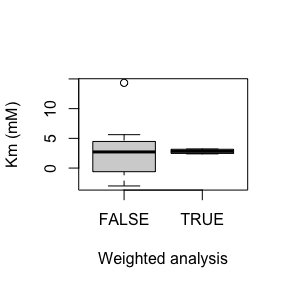## Hanes-Woolf Multiplying both sides of the equation 1 by [S] we reach: $\begin{equation} \tag{2} \frac{[S]}{v} = \frac{1}{V_{max}} [S] + \frac{K_m}{V_{max}} \end{equation}$ The function hw() takes as argument the original data $$([S], v)$$ and transform them to $$([S], \frac{[S]}{v})$$ and afterwards it fits these transformed variables to the equation of a line to estimate the kinetic parameters. Let’s illustrate the use of this function with the data from group 7: hw7 <- hw(ONPG[ , c(1,8)], unit_v = 'uM/min')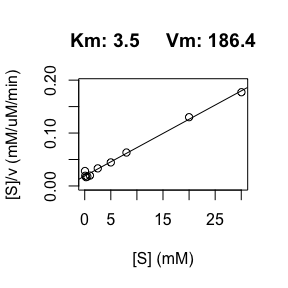After all, the data generated by the group 7 do not seem as bad as we might have believed when we analyze them using unweighted double-reciprocal analysis. ## Eadie-Hofstee Multiplying both sides of the equation 1 by $$v V_{max}$$ we reach: $\begin{equation} \tag{3} v = -K_m \frac{v}{[S]} + V_{max} \end{equation}$ We will use again data from group 7 to carry out the analysis: eh7 <- eh(ONPG[ , c(1,8)], unit_v = 'uM/min')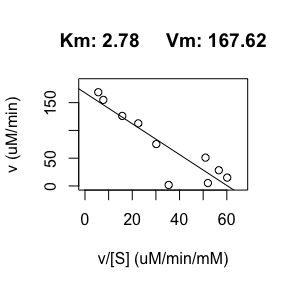## Eisenthal & Cornish-Bowden Finally we will illustrate this alternative method using again the original data from group 7. ecb7 <- ecb(ONPG[ , c(1,8)], unit_v = "uM/min")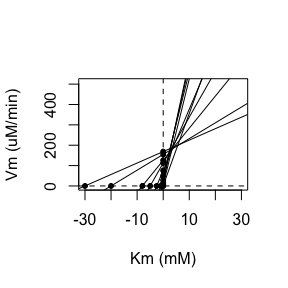ecb7$Km
#>  "Km:  2.857  ±  NA   mM"
ecb7\$Vm
#>  "Vm:  261.505  ±  NA   uM/min"